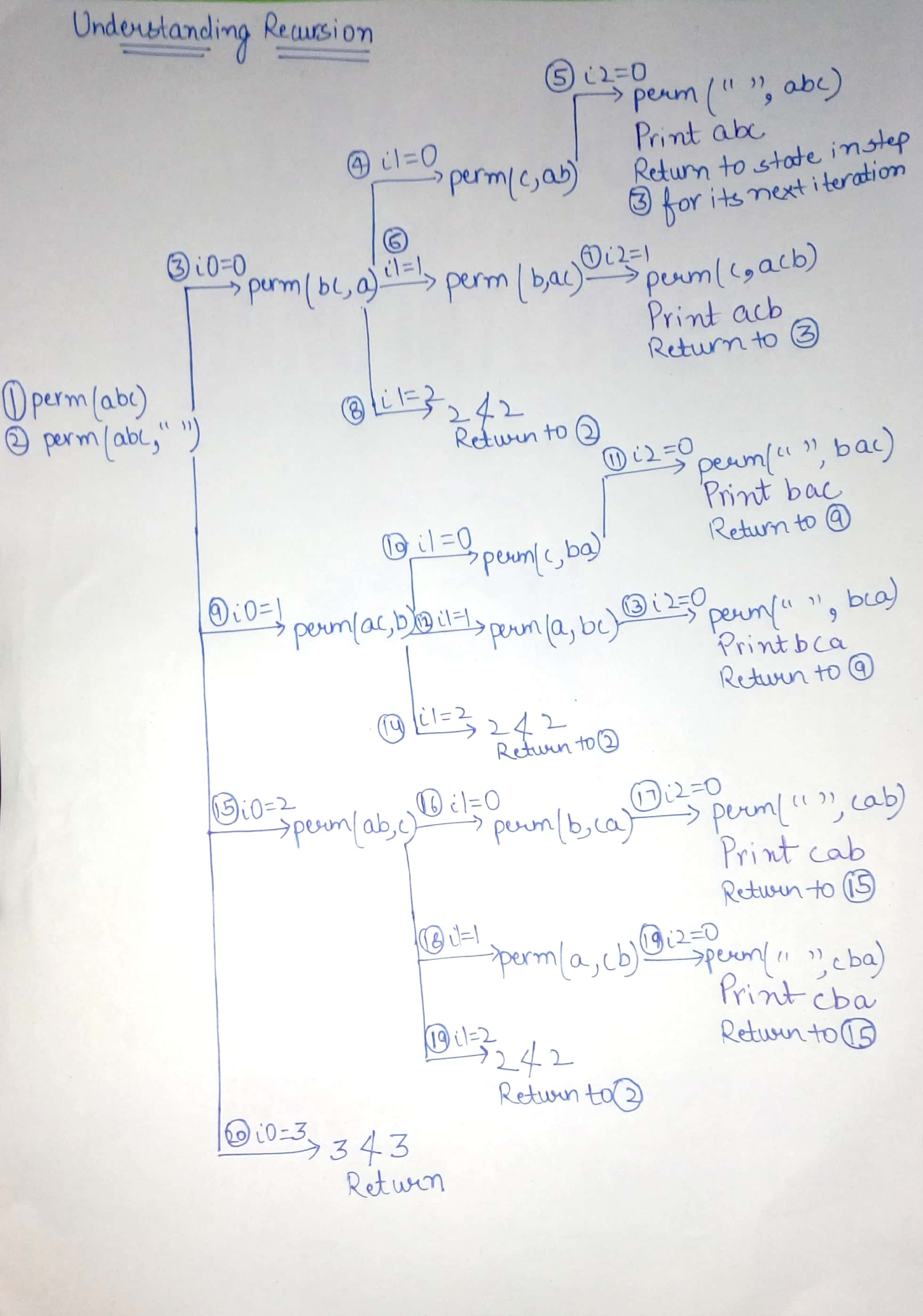# Time complexity of all permutations of a string

Time complexity of printing all permutations of a string.

```1. void perm(String str){
2.    perm(str, "");
3.  }
4.
5. void perm(String str, String prefix){
6.     if(str.length() == 0){
7.         System.out.println(prefix);
8.     } else{
9.        for(int i = 0; i < str.length(); i++){
10.           String rem = str.substring(0, i) +
str.substring(i + 1);
11.           perm(rem, prefix + str.charAt(i));
12.       }
13.    }
14. }
```

Understanding the Code:

Line 1-3 : When call is to function perm passing “abc” as string argument, an internal call to perm(str, “”) is made. This means that it is starting with an empty prefix (second argument) to create permutations for the entire string (first argument).

Line 6-8 : This is the base case that will stop recursion. If there are no more characters left to be permuted in the input string, then print current permutation held in variable prefix and return.

Line 9-12 : The for loop picks one character from input string at a time to update prefix string. That is, loop makes a call to function perm again with updated prefix and another string rem which collects the remaining characters of the input string.

Understand this with the help of following image :Analyzing the Time Complexity :

1. How many times does function perm get called in its base case?
As we can understand from the recursion explained above that for a string of length 3 it is printing 6 permutations which is actually 3!. This is because if it needs to generate permutation, it is needed to pick characters for each slot. If there are 3 characters in our string, in the first slot, there are 3 choices, 2 choices for the next slot (for each of 3 choices earlier, i.e multiplication and not addition) and so on. This tells that there are n! permutations being printed in the base case which is what is shown in the image.

2. How many times does function perm get called before its base case?
Consider that lines 9 through 12 are hit n number of times. Therefore, there will be no more than (n * n!) function calls.

3. How long does each function call take?
Since, each character of string prefix needs to be printed, thus executing line 7 will take O(n) time. Line 10 and line 11 will also take O(n) time combined due to string concatenation, as sum of rem, prefix and str.charAt(i) will always be n. Each function call therefore corresponds to O(n) work.

4. What is the total runtime?
Calling perm O(n * n!) times (as an upper bound) and each call takes O(n) time, the total runtime will not exceed O(n^2 * n!).

Source: Cracking the Coding Interview by Gayle Laakmann McDowell

Attention reader! Don’t stop learning now. Get hold of all the important DSA concepts with the DSA Self Paced Course at a student-friendly price and become industry ready.

My Personal Notes arrow_drop_upCheck out this Author's contributed articles.

If you like GeeksforGeeks and would like to contribute, you can also write an article using contribute.geeksforgeeks.org or mail your article to contribute@geeksforgeeks.org. See your article appearing on the GeeksforGeeks main page and help other Geeks.

Please Improve this article if you find anything incorrect by clicking on the "Improve Article" button below.

Article Tags :
Practice Tags :

2

Please write to us at contribute@geeksforgeeks.org to report any issue with the above content.